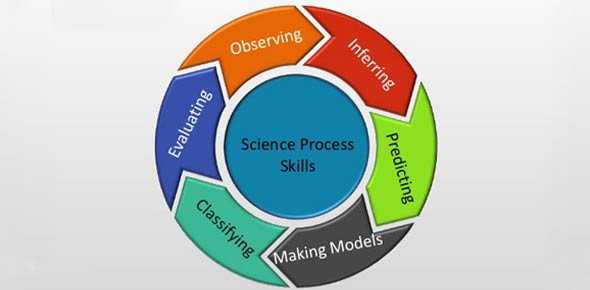# 7th Quiz Science Process Skills

15 Questions | Total Attempts: 295Settings• 1.
Of the following which is a qualitative observation
• A.

It is blue

• B.

It is 5 cm long

• C.

I like the bue color

• D.

It has a mass of 10.1g

• 2.
Of the following which is a quantitative observation
• A.

It is 2mm in length

• B.

It has a mass of 505 kg

• C.

It has a density 5.3 g/cm cubed

• D.

It is very soft

• 3.
To find the density of an object you will need to know the mass and the
• A.

Volume

• B.

Weight

• C.

Length

• D.

Grams

• 4.
The scientific method always begins with some sort of
• A.

• B.

An experiment

• C.

A hypothesis

• D.

Conclusions

• 5.
The traditional scientific method is basically linear starting with the Question and ending with the...
• A.

Conclusion

• B.

Experiment

• C.

Observations

• D.

Try agains

• 6.
In an experiment there are many factors that change, we refer to these as different types of
• A.

Variables

• B.

Questions

• C.

Experiments

• D.

• 7.
Making conversions within the metric system is easy.  All you have to do is ...
• A.

Move the decimal the proper number of spaces

• B.

Multiply by the conversion factor

• C.

Subtract by the number of steps you take

• D.

Add the number of steps you take

• 8.
Using body parts for a measurement system is...
• A.

A bad idea because everyone is different sizes

• B.

A great idea because everyone carries measuring tools around with them

• C.

A great idea because it makes measuring personal

• D.

Still used by most countries in the world

• 9.
When measuring the amount of liquid in a graduated cylinder you should always
• A.

• B.

Hold it up to the light

• C.

Read it from the bottom up

• D.

• 10.
Which of the following is the base unit for length in the metric system
• A.

Meter

• B.

Liter

• C.

Gram

• D.

Inch

• 11.
0.81 g =_____ cg
• A.

81

• B.

8.1

• C.

0.0081

• D.

2.81

• 12.
4.6 Km = _____m
• A.

4,600

• B.

0.046

• C.

0.46

• D.

460

• 13.
218 cm = _____ Km
• A.

0.00218

• B.

21,800

• C.

0.218

• D.

2.18

• 14.
3.5 l = ______ ml
• A.

3,500

• B.

35

• C.

350

• D.

0.035

• 15.
The independent variable in an experiment is the variable that
• A.

You intentionally change

• B.

You can have 3 or 4 in an experiment

• C.

Responds to what you change

• D.

Stays the same no matter what

Related TopicsBack to top